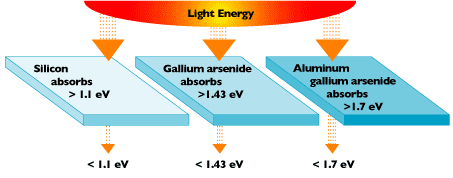A# band gapDifferent photovoltaic materials have different energy band gaps. Photons with energy equal to the band gap energy are absorbed to create free electrons. Photons with less energy than the band gap energy pass through the material.

In a semiconductor, such as silicon, the band gap is the energy difference between the highest valence band and the lowest conduction band. The band gap energy is the amount of energy (in electron volts) required to free an outer shell electron from its orbit about the nucleus to a free state, and thus promote it from the valence to the conduction level.

## Band gaps and solar cells

To free an electron, the energy of a photon must be at least as great as the band gap energy. However, photons with more energy than the band gap energy will expend that extra amount as heat when freeing electrons. So, it's important for a solar cell to be "tuned" – through slight modifications to the silicon's molecular structure – to optimize the photon energy. A key to obtaining an efficient solar cell is to convert as much sunlight as possible into electricity.

Crystalline silicon has a band gap energy of 1.1 electron-volts (eV). (An electron-volt is equal to the energy gained by an electron when it passes through a potential of 1 volt in a vacuum.) The band gap energies of other effective photovoltaic semiconductors range from 1.0 to 1.6 eV. In this range, electrons can be freed without creating extra heat.

The photon energy of light varies according to the different wavelengths of the light. The entire spectrum of sunlight, from infrared to ultraviolet, covers a range of about 0.5 eV to about 2.9 eV. For example, red light has an energy of about 1.7 eV, and blue light has an energy of about 2.7 eV. Most solar cells cannot use about 55% of the energy of sunlight, because this energy is either below the bandgap of the material or carries excess energy.